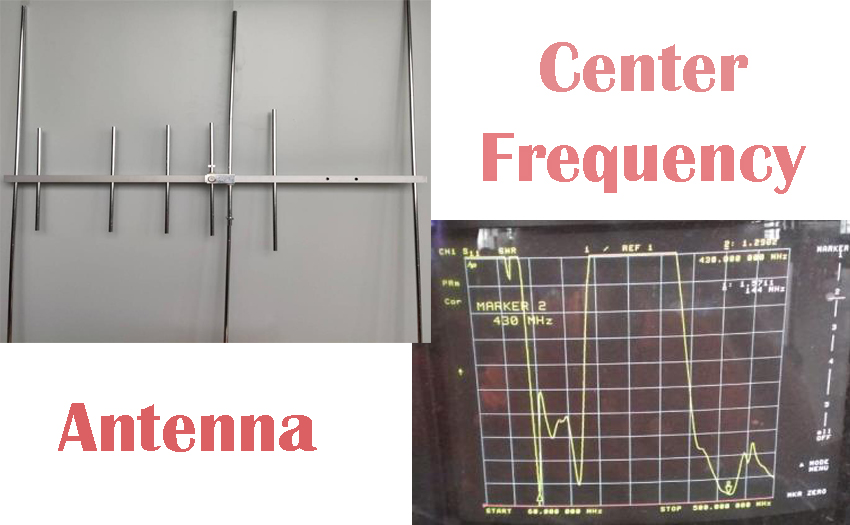# What's the center frequency of antennaThe basic definition of the center frequency refers to the geometric mean of the bandpass or bandstop filter frequency. In logarithmic coordinates, it is the midpoint between two 3dB points. It is generally represented by the arithmetic average of the two 3dB points.

Each antenna has a certain frequency range called bandwidth. In the range, the antenna has the lower impedance and the highest efficiency, which is equivalent to a band-pass filter. The best frequency point in the middle of the frequency range is the center frequency, its SWR is the lowest, and the efficiency is higher. For example, Ailunce AY04 is a dual-band yagi antenna. Its frequency range is UHF&VHF, that is said it can work at all the frequencies in the range, but it also has the center frequency both in the UHF and VHF bands. The center frequency of VHF is 144MHZ and the center frequency of UHF is 430MHZ. It's the reason that we recommend that customers choose the frequency point close to the center frequency when they want to obtain the highest output power.

Using the feature of the smallest SWR of the center frequency, we can measure the center frequency of the antenna with a known preset frequency range. When measuring, we should try to widen the frequency range of testing, so that the lowest point will be more obvious.